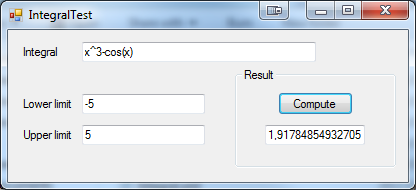### Features And Algorithms

This library uses numerical calculator library so can handle same functions at input.

To compute integrals library uses ten Gauss–Legendre quadratures. Thanks to this computations are very fast and accurate.

### Usage

`string formula = "x ^ 3 - cos(x)";double lowerLimit = -5d;double upperLimit = 5d;Integral integral = new Integral(formula, lowerLimit, upperLimit);double result = integral.ComputeIntegral();`

### Example application